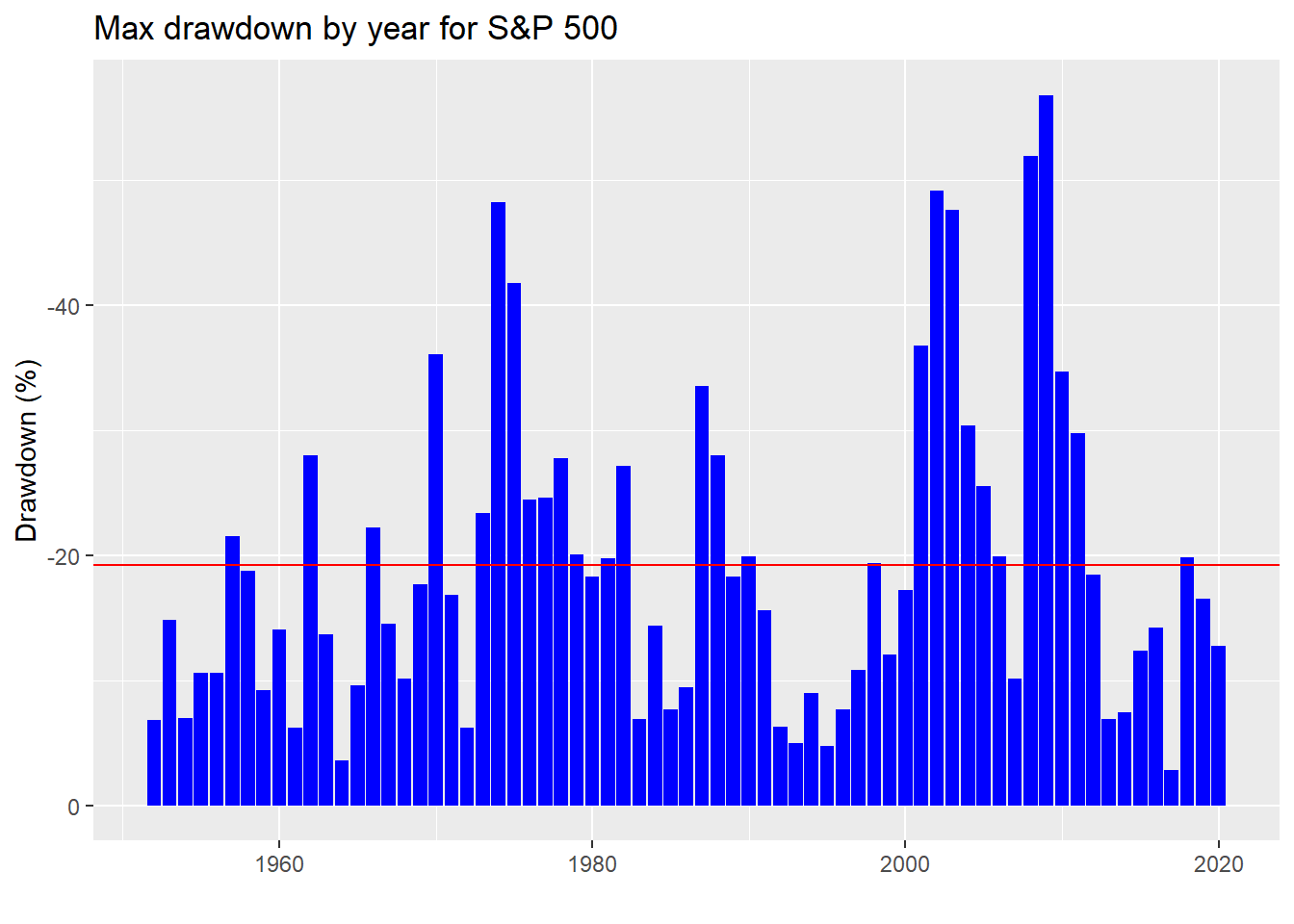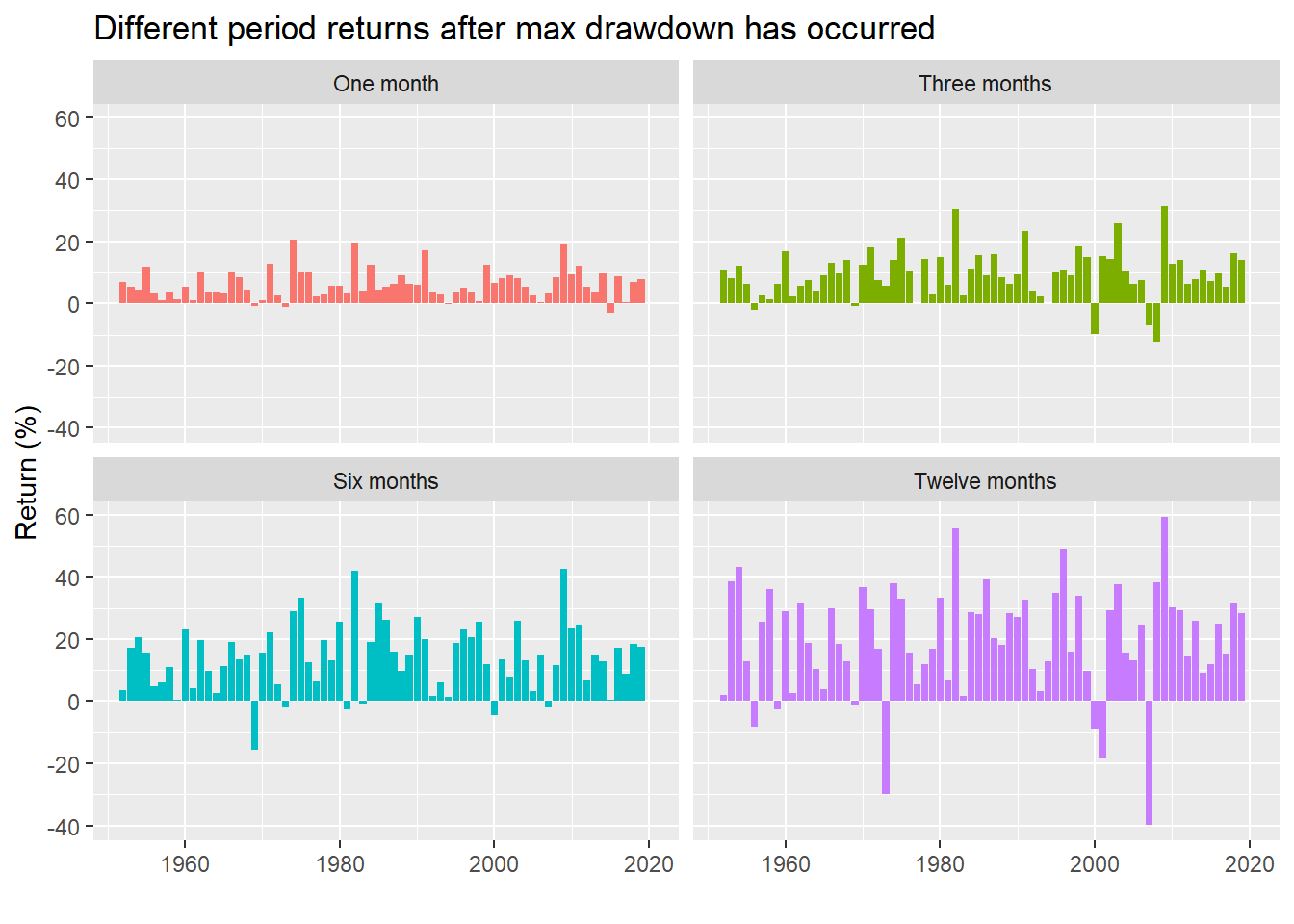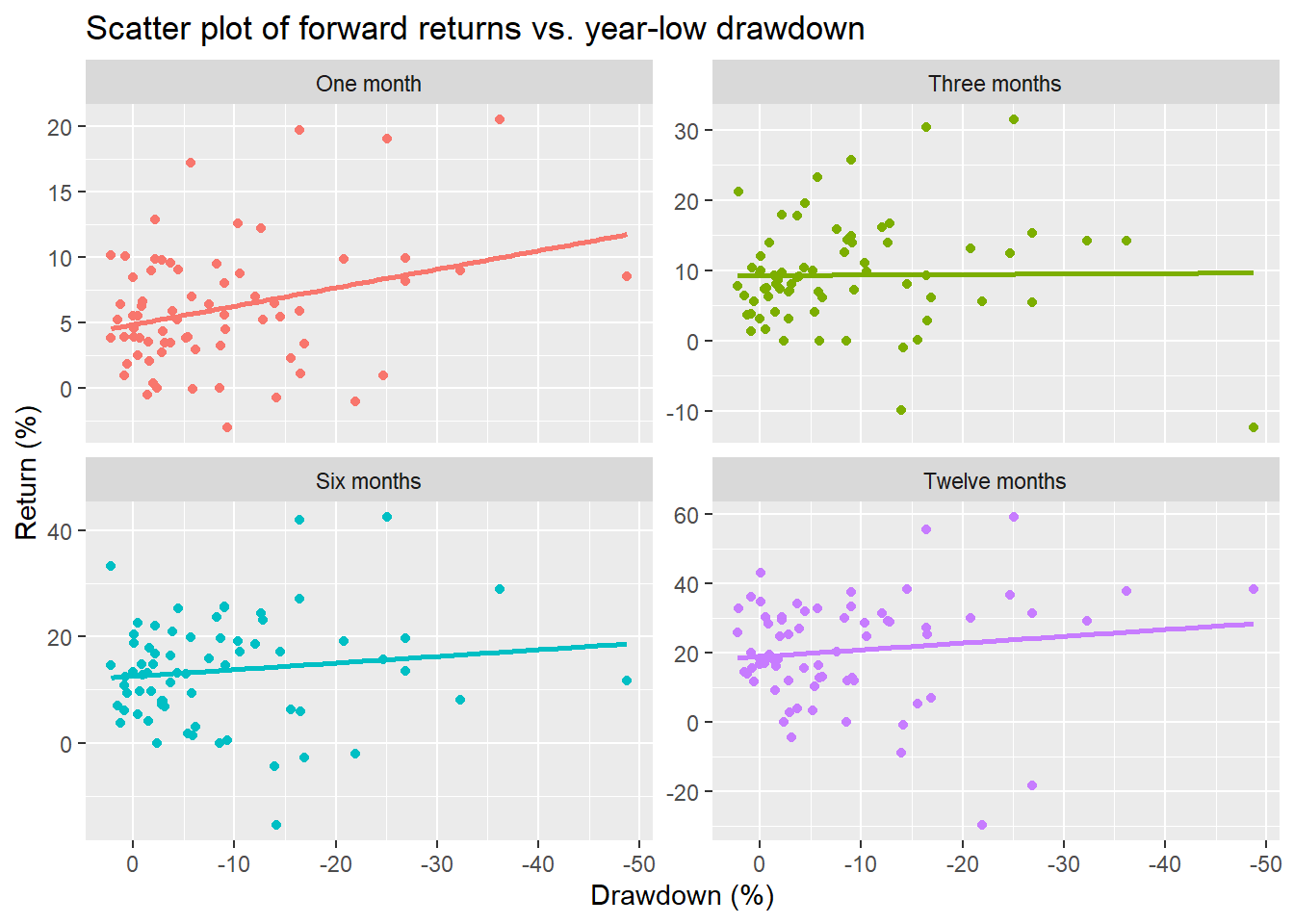Want to share your content on R-bloggers? click here if you have a blog, or here if you don't.

We’re taking a break from our series on portfolio construction for two reasons: life and the recent market sell-off. Life got in the way of focusing on the next couple of posts on rebalancing. And given the market sell-off we were too busy gamma hedging our convexity exposure, looking for cheap tail risk plays, and trying to figure out when we should go long the inevitable vol crush. Joking. We’re not even sure what any of that means. But we do know that applying data science to the recent market turbulence may provide some perspective.

As of the close of the US market on 2/28, the S&P 500 and many other indices were already in correction mode, which is typically defined as a 10% decline from the most recent peak. The cause for the sell-off was relatively clear: the global spread of the coronavirus. What’s not is precisely why the market decided now was the time to discount the global impact. We have no insight on that. But we can offer an historical perspective on drawdowns through the aid of R programming and data science. This post is mainly focused on data exploration. If you’re expecting predictions, we predict with absolute confidence the market will be volatile over the next three years! Finance humor never gets old.

First, some perspective. Markets are volatile; thus there’s going to be a drawdown of some magnitude every year. Indeed, Peter Lynch, the famous portfolio manager of Fidelity’s one-time flagship Magellan fund, was quoted as saying something akin to there’s a 50% chance of a 10% decline or more in any year. With that comment as motivation, let’s begin.

We start by pulling the S&P 500 data and looking at the maximum drawdown from the previous peak of the past 252-day trading period. We then calculate the current return from that peak, selecting the minimum return to arrive at the maximum drawdown of the year. We then average the max drawdown across years, which is the red line in the graph is below. We flip the y-axis so that one can easily distinguish the greatest drawdown as the tallest column.Lynch’s probability of a 10% or greater drawdown was based on a mixture of indices1 that included 95 years of data. Our data set, which begins with the inception of the S&P 500, is based on 70 years of data. In this case, there’s a 73.9% chance of seeing a decline of greater than 10% in any year. It wasn’t clear which reference point Lynch was using so we’ll also look at drawdowns vs the beginning of the year—in other words, the yearly low. In this case, we get a slightly less ominous picture as seen below.In fact, basing the probabilities on end of year closes we calculate that a drawdown of greater than 10% occurs only 31.4% time. Which start date is more important? It depends.

For most active managers, performance metrics reset at the beginning of the year, so a greater than 10% drawdown from the end of the previous year would no doubt be on their minds. However, in an upwardly trending market, the low point of the year may not be the point of the largest drawdown—the so-called “higher highs and higher lows” effect. Hence, the impact of a larger drawdown from a higher level might not be as psychologically draining unless it ends up yielding a down year or underperformance.

Whatever the case, while we can make arguments for both, let the data do the talking. First, we’ll calculate forward returns starting from the day after the max drawdown occurred and ending 22, 66, 126, and 252 trading days later, equivalent to one, three, six, and twelve month returns. The following is the graph for the returns based on peak-to-trough max drawdown.While this is clearly a lot of data, let’s shrink it a bit and see how often there was a positive return in the following period. In other words, what was the win rate?

Table 1: Percent of positive returns by period
Period Win rate (%)
One month 94.2
Three months 91.3
Six months 91.3
Twelve months 89.9

Pretty much 90%. Not bad. How about calculating returns based on the low point of the year?Not too different. What about win rate?

Table 2: Percent of positive returns by period
Period Win rate (%)
One month 92.9
Three months 94.3
Six months 94.3
Twelve months 92.9

Slightly better. Let’s look at some scatter plots of the drawdowns vs forward returns. We’ll include a regression line and reverse the direction of the x-axis to highlight the relationship.As we can see there’s a linear relationship between the magnitude of the drawdown and returns over the next few periods. But it’s not extreme. That is, the gradient of the line isn’t that high. Let’s regress the various period returns against the drawdowns to see what the effects might be. We present the regression output below.

Table 3: Regression output for peak-to-trough drawdown
Period Size effect T-statistic R-squared
One month -0.18 -4.2 0.21
Three months -0.21 -3.0 0.12
Six months -0.33 -3.3 0.14
Twelve months -0.48 -2.9 0.11

As we can see from the regression statistics, the size effect is modest, much less than one for one. Nonetheless, the effect is significant based on the t-statistics, though the overall explanatory power (not unexpectedly) is low based on the r-squared.

Let’s now look at the scatter plot based on yearly low drawdowns.The linear relationship appears to be more modest than the peak-to-trough drawdown.

Table 4: Regression output of year-low drawdown
Period Size effect T-statistic R-squared
One month -0.14 -2.5 0.09
Three months -0.01 -0.1 0.00
Six months -0.13 -1.0 0.02
Twelve months -0.19 -1.1 0.02

The effect of the yearly low drawdown on succeeding returns is not as significant as the peak-to-trough. Interestingly, the size effect on three-month returns has almost no signficance. On first blush, we’re not sure why that might be the case other than when the low falls with respect to the time of the year. But we’ll shelve that for now. Predictably, the r-squareds are low too.

While a host of factors can be linked to any single drawdown, one wonders whether larger sell-offs see a greater rebound (if any). Given that we’re using continuous variables, we’ll need to bucket the returns to compare them. We’ll transform the drawdowns into categorical variables based on quintiles and present the mean return for the 22 to 252-day periods we looked at above.

Table 5: Average returns (%) grouped by quintile drawdown (%)
Quantile Obs. Drawdown One month Three months Six months Twelve months
First 5 -50.7 13.0 14.6 23.3 40.4
Second 3 -38.2 6.4 16.4 20.8 17.1
Third 11 -28.5 8.2 11.7 17.3 22.3
Fourth 24 -17.9 5.9 9.1 13.2 21.5
Fifth 26 -8.1 4.0 6.6 9.9 12.3

We see that the larger drawdown (as encoded by the lower order quantile) yields a higher return in most cases. While it would be nice to test the significance of these means, we see that the number of observations (Obs. column) is relatively low, so the results wouldn’t be robust in many cases. Nonetheless, the fourth and fifth quintiles appear to have sufficient data. When we run a t-test on the twelve-month returns, there’s only a 5.9% chance the differences are due to randomness. While not at the 5% significance level most like to use, it is pretty close. Hence, there is an argument that the larger the drawdown, the greater the rebound, but it is not exceedingly strong given the limited data. If we arbitrarily split the data at the fourth quintile, we find the significance improves to 1.1%.

We’ll end it here with a few takeaways. Peak-to-trough drawdowns appear to be more robust than yearly low drawdowns in estimating the resulting bounce. But sell-offs only describe a portion of the variability of returns going forward given the low r-squareds. There is some evidence that higher magnitude drawdowns yield higher succeeding rebounds, but selecting robust break points is difficult given the limited observations of high absolute declines. When we use a simple cut-off, the significance improves.

If there’s more interest in more detailed analysis of drawdowns, shoot us an email at nbw dot osm at gmail dot com and we’ll look to expand this post. Until next time, here’s the code behind the above analysis.

```# load packages
library(tidyquant)
library(tidyverse)

sp <- getSymbols("^GSPC", src = "yahoo", from = "1950-01-01", auto.assign = FALSE) %>%
`colnames<-`("sp")

# Create drawdowm vector
n_row <- nrow(sp)
draw_downs <- c()
maxs <- c()
max_p <- 0
for(i in 252:n_row){
max_p <- max(sp[(i-251):i], max_p)
draw_downs[i] <- sp[i]/max_p-1
maxs[i] <- max_p
}

# Create drawdown from end of year
sp_yr <- to.yearly(sp)
end_dd <- Lo(to.yearly(sp))/Lag(Cl(to.yearly(sp)))-1

# Create dataframe
df <- data.frame(date = index(sp), dd = draw_downs)
df_end <- data.frame(date = index(end_dd), dd = as.numeric(end_dd))

# Find mean of max drawdown
mean_dd <-df %>%
mutate(year = year(date)) %>%
group_by(year) %>%
summarize(max_dd = min(dd)) %>%
summarize(mean_dd = mean(max_dd, na.rm = TRUE)) %>%
as.numeric()

mean_dde <-df_end %>%
mutate(year = year(date)) %>%
group_by(year) %>%
summarize(max_dd = min(dd)) %>%
summarize(mean_dd = mean(max_dd, na.rm = TRUE)) %>%
as.numeric()

# Graph max drawdown by year
df %>% mutate(year = year(date)) %>%
group_by(year) %>%
summarize(max_dd = min(dd)) %>%
ggplot(aes(year, max_dd*100)) +
geom_bar(stat = "identity", fill = "blue") +
geom_hline(yintercept = mean_dd*100, color = "red") +
scale_y_reverse()+
labs(x = "",
y = "Drawdown (%)",
title = "Max drawdown by year for S&P 500")

# Count drawdowns greater than 10%
probs <- df %>%
mutate(year = year(date)) %>%
group_by(year) %>%
summarize(max_dd = min(dd)) %>%
summarize(round(mean(max_dd < -0.1, na.rm = TRUE),3)*100) %>%
as.numeric()

probs_end <- df_end %>%
mutate(year = year(date)) %>%
group_by(year) %>%
summarize(max_dd = min(dd)) %>%
summarize(round(mean(max_dd < -0.1, na.rm = TRUE),3)*100) %>%
as.numeric()

# Find mean drawdown
mean_dde <-df_end %>%
mutate(year = year(date)) %>%
group_by(year) %>%
summarize(max_dd = min(dd)) %>%
summarize(mean_dd = mean(max_dd, na.rm = TRUE)) %>%
as.numeric()

# Graph max drawdown by year
df_end %>% mutate(year = year(date)) %>%
group_by(year) %>%
summarize(max_dd = min(dd)) %>%
ggplot(aes(year, max_dd*100)) +
geom_bar(stat = "identity", fill = "blue") +
geom_hline(yintercept = mean_dde*100, color = "red") +
scale_y_reverse()+
labs(x = "",
y = "Drawdown (%)",
title = "Max drawdown from prior year close by year for S&P 500")

# Count drawdowns greater than 10%
probs_end <- df_end %>%
mutate(year = year(date)) %>%
group_by(year) %>%
summarize(max_dd = min(dd)) %>%
summarize(round(mean(max_dd < -0.1, na.rm = TRUE),3)*100) %>%
as.numeric()

# Find max drawdowns

# Yearly high
df1 <- df %>%
separate(date,c("year", "month", "day"), sep = "-") %>%
group_by(year) %>%
filter(dd == min(dd)) %>%
ungroup() %>%
unite("date", year:day, sep = "-") %>%
mutate(date = ymd(date))

# Beginning of the year
sp_df <- data.frame(date = index(sp), sp = as.numeric(sp[,1]))

lows <- sp_df %>%
separate(date, c("year", "month", "day"), sep = "-") %>%
group_by(year) %>%
filter(sp == min(sp)) %>%
ungroup() %>%
unite("date", year:day, sep = "-") %>%
mutate(date = ymd(date))

df_end <- df_end %>%
mutate(date = lows\$date) %>%
na.omit()

# Find forward returns
idx <- which(index(sp) %in% df1\$date) + 1
steps <- c("22", "66", "126", "252")
ret <- list()
for(step in steps){
ret[[step]] <- as.numeric(sp[idx[-length(idx)]+as.numeric(step)])/as.numeric(sp[idx[-length(idx)]])-1
}

rets <- ret %>% bind_cols()
rets_names <- paste("t", steps, sep = "_")
names(rets) <- rets_names
rets <- rbind(rets,rep(0,4))

# Find forward returns on low of year
idx1 <- which(index(sp) %in% df_end\$date) + 1
ret1 <- list()
for(step in steps){
ret1[[step]] <- as.numeric(sp[idx1[1:(length(idx1)-2)]+as.numeric(step)])/as.numeric(sp[idx1[1:(length(idx1)-2)]])-1
}

rets1 <- ret1 %>% bind_cols()
rets_names <- paste("t", steps, sep = "_")
names(rets1) <- rets_names
rets1 <- rbind(rets1,rep(0,4), rep(0,4))

# Add forward returns to df1
df1 <- df1 %>% bind_cols(rets)

# Add forward returns to df_end
df_end <- df_end %>% bind_cols(rets1)

# Graph
df1 %>%
mutate(date = year(date)) %>%
gather(key, value, -c(date,dd)) %>%
mutate(key = factor(key, levels = c("t_22", "t_66", "t_126", "t_252"))) %>%
ggplot(aes(date, value*100, fill = key)) +
geom_bar(stat = "identity") +
facet_wrap(~key,
# scales = "free_y",
labeller = as_labeller(c(t_22 = "One month",
t_66 = "Three months",
t_126 = "Six months",
t_252 = "Twelve months"))) +
# scale_fill_manual("", values = c("slategrey", "red", "purple", "blue")) +
theme(legend.position = "none") +
labs(x= "",
y = "Return (%)",
title = "Different period returns after max drawdown has occurred")

df1 %>%
gather(key, value, -c(date,dd)) %>%
mutate(key = factor(key, levels = c("t_22", "t_66", "t_126", "t_252"))) %>%
group_by(key) %>%
summarise(prob = round(mean(value >= 0),3)*100) %>%
mutate(key = case_when(key == "t_22" ~"One month",
key == "t_66"~"Three months",
key == "t_126"~"Six months",
key == "t_252"~"Twelve months")) %>%
rename("Period" = key,
"Win rate (%)" = prob) %>%
knitr::kable(caption = "Percent of positive returns by period")

# Year graph
df_end %>%
mutate(date = year(date)) %>%
gather(key, value, -c(date,dd)) %>%
mutate(key = factor(key, levels = c("t_22", "t_66", "t_126", "t_252"))) %>%
ggplot(aes(date, value*100, fill = key)) +
geom_bar(stat = "identity") +
facet_wrap(~key,
# scales = "free_y",
labeller = as_labeller(c(t_22 = "One month",
t_66 = "Three months",
t_126 = "Six months",
t_252 = "Twelve months"))) +
# scale_fill_manual("", values = c("slategrey", "red", "purple", "blue")) +
theme(legend.position = "none") +
labs(x= "",
y = "Return (%)",
title = "Different period returns after max drawdown has occurred")

# count positive returns
df_end %>%
gather(key, value, -c(date,dd)) %>%
mutate(key = factor(key, levels = c("t_22", "t_66", "t_126", "t_252"))) %>%
group_by(key) %>%
summarise(prob = round(mean(value >= 0),3)*100) %>%
mutate(key = case_when(key == "t_22" ~"One month",
key == "t_66"~"Three months",
key == "t_126"~"Six months",
key == "t_252"~"Twelve months")) %>%
rename("Period" = key,
"Win rate (%)" = prob) %>%
knitr::kable(caption = "Percent of positive returns by period")

# Scatter plot
df1 %>%
gather(key, value, -c(date, dd)) %>%
mutate(key = factor(key, levels = c("t_22", "t_66", "t_126", "t_252"))) %>%
ggplot(aes(dd*100, value*100, color = key)) +
geom_point() +
geom_smooth(method = "lm", se = FALSE) +
scale_x_reverse() +
facet_wrap(~key,
scales = "free_y",
labeller = as_labeller(c(t_22 = "One month",
t_66 = "Three months",
t_126 = "Six months",
t_252 = "Twelve months"))) +
labs(x = "Drawdown (%)",
y = "Return (%)",
title = "Scatter plot of forward returns vs. peak-to-trough drawdown") +
theme(legend.position = "none")

# Regression
reg_out <- data.frame(period = rets_names,
size_eff = rep(0,4),
t_stats = rep(0,4),
rsq = rep(0,4))

for(name in rets_names){
form <- as.formula(paste(name, "dd", sep = "~"))
reg_out[reg_out\$period == name,2] <- df1 %>%
lm(form, .) %>%
broom::tidy() %>%
filter(term != "(Intercept)") %>%
.\$estimate %>%
round(., 2)

reg_out[reg_out\$period == name,3] <- df1 %>%
lm(form, .) %>%
broom::tidy() %>%
filter(term != "(Intercept)") %>%
.\$statistic %>%
round(., 1)

reg_out[reg_out\$period == name,4] <- df1 %>%
lm(form, .) %>%
broom::glance() %>%
.\$r.squared %>%
round(.,2)

}

reg_out %>%
mutate(period = case_when(period == "t_22" ~"One month",
period == "t_66"~"Three months",
period == "t_126"~"Six months",
period == "t_252"~"Twelve months")) %>%
rename("Period" = period,
"T-statistic" = t_stats,
"Size effect" = size_eff,
"R-squared" = rsq) %>%
knitr::kable(caption = "Regression output for peak-to-trough drawdown")

# Scatter plot
df_end %>%
gather(key, value, -c(date, dd)) %>%
mutate(key = factor(key, levels = c("t_22", "t_66", "t_126", "t_252"))) %>%
ggplot(aes(dd*100, value*100, color = key)) +
geom_point() +
geom_smooth(method = "lm", se = FALSE) +
scale_x_reverse() +
facet_wrap(~key,
scales = "free_y",
labeller = as_labeller(c(t_22 = "One month",
t_66 = "Three months",
t_126 = "Six months",
t_252 = "Twelve months"))) +
labs(x = "Drawdown (%)",
y = "Return (%)",
title = "Scatter plot of forward returns vs. year-low drawdown") +
theme(legend.position = "none")

# Regression
reg_out1 <- data.frame(period = rets_names,
size_eff = rep(0,4),
t_stats = rep(0,4),
rsq = rep(0,4))

for(name in rets_names){
form <- as.formula(paste(name, "dd", sep = "~"))
reg_out1[reg_out1\$period == name,2] <- df_end %>%
lm(form, .) %>%
broom::tidy() %>%
filter(term != "(Intercept)") %>%
.\$estimate %>%
round(., 2)

reg_out1[reg_out1\$period == name,3] <- df_end %>%
lm(form, .) %>%
broom::tidy() %>%
filter(term != "(Intercept)") %>%
.\$statistic %>%
round(., 1)

reg_out1[reg_out1\$period == name,4] <- df_end %>%
lm(form, .) %>%
broom::glance() %>%
.\$r.squared %>%
round(.,2)

}

reg_out1 %>%
mutate(period = case_when(period == "t_22" ~"One month",
period == "t_66"~"Three months",
period == "t_126"~"Six months",
period == "t_252"~"Twelve months")) %>%
rename("Period" = period,
"Size effect" = size_eff,
"T-statistic" = t_stats,
"R-squared" = rsq) %>%
knitr::kable(caption = "Regression output of year-low drawdown")

df1 %>%
mutate(quant = cut_interval(dd,5,
labels = c("First", "Second", "Third", "Fourth", "Fifth"))) %>%
group_by(quant) %>%
mutate(count = n()) %>%
group_by(quant, count) %>%
select(-c(date, dd)) %>%
summarise_all(function(x) round(mean(x),3)*100) %>%
rename("Quantile" = quant,
"Obs." = count,
"One month" = t_22,
"Three months" = t_66,
"Six months" = t_126,
"Twelve months" = t_252) %>%
knitr::kable()

# T-test
t_test <- df1 %>%
mutate(quant = cut_interval(dd,5,
labels = c("First", "Second", "Third", "Fourth", "Fifth"))) %>%
group_by(quant) %>%
filter(quant %in% c("Fourth", "Fifth")) %>%